# NCERT Solutions for Class 7 Maths Chapter 15 Visualising Solid Shapes (EX 15.2) Exercise 15.2

## NCERT Solutions for Class 7 Maths Chapter 15 Visualising Solid Shapes (EX 15.2) Exercise 15.2

Free PDF download of NCERT Solutions for Class 7 Maths Chapter 15 Exercise 15.2 (EX 15.2) and all chapter exercises at one place prepared by expert teacher as per NCERT (CBSE) books guidelines. Class 7 Maths Chapter 15 Visualising Solid Shapes Exercise 15.2 Questions with Solutions to help you to revise complete Syllabus and Score More marks. Register and get all exercise solutions in your emails.

Do you need help with your Homework? Are you preparing for Exams?
Study without Internet (Offline)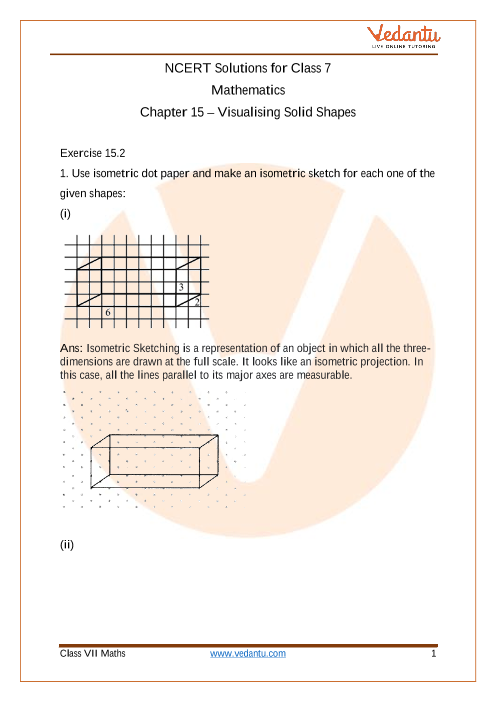Book your Free Demo session
Get a flavour of LIVE classes here at Vedantu## Access NCERT Solutions for Class 7 Chapter 15 – Visualising Solid Shapes

Exercise 15.2

1. Use isometric dot paper and make an isometric sketch for each one of the

given shapes:

(i)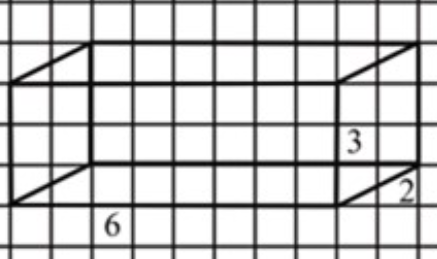Ans: Isometric Sketching is a representation of an object in which all the three-dimensions are drawn at the full scale. It looks like an isometric projection. In this case, all the lines parallel to its major axes are measurable.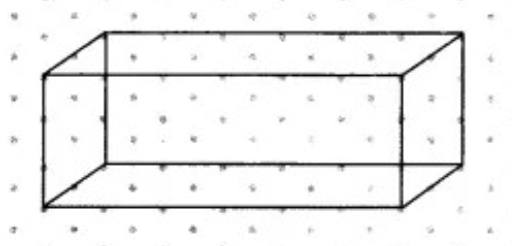(ii)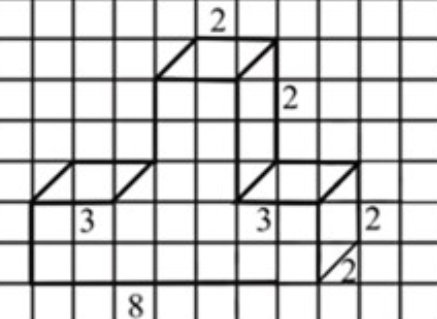Ans: Isometric Sketching is a representation of an object in which all the three-dimensions are drawn at the full scale. It looks like an isometric projection. In this case, all the lines parallel to its major axes are measurable.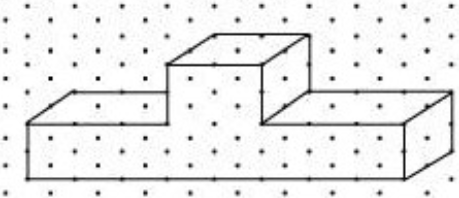(iii)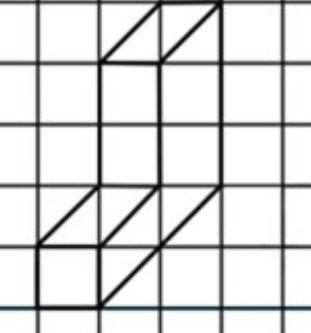Ans: Isometric Sketching is a representation of an object in which all the three-dimensions are drawn at the full scale. It looks like an isometric projection. In this case, all the lines parallel to its major axes are measurable.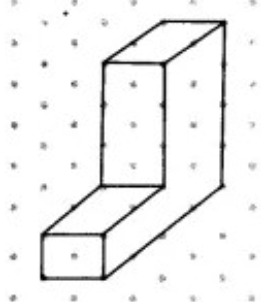(iv)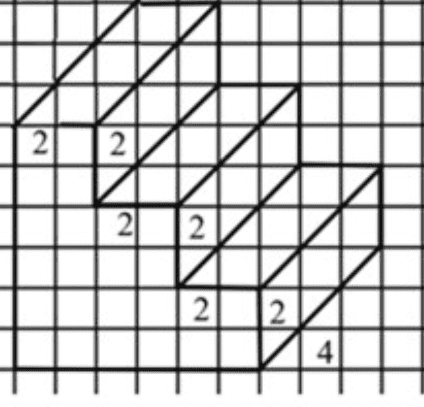Ans: Isometric Sketching is a representation of an object in which all the three-dimensions are drawn at the full scale. It looks like an isometric projection. In this case, all the lines parallel to its major axes are measurable.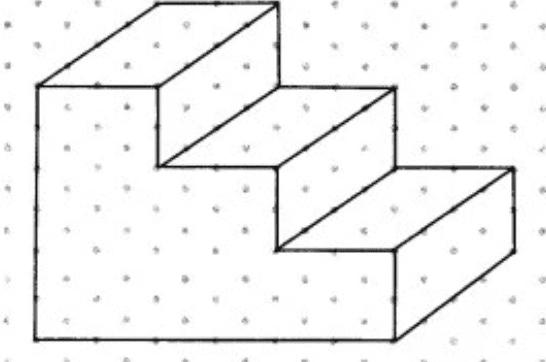2. The dimensions of a cuboid are 5 cm, 3 cm and 2 cm. Draw three different isometric sketches of this cuboid.

Ans: Isometric Sketching is a representation of an object in which all the three-dimensions are drawn at the full scale. It looks like an isometric projection. In this case, all the lines parallel to its major axes are measurable.

The three different isometric sketches of this cuboid having dimension 5 cm, 3 cm and 2 cm is shown below,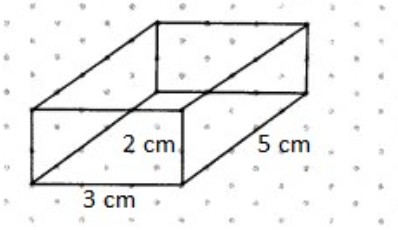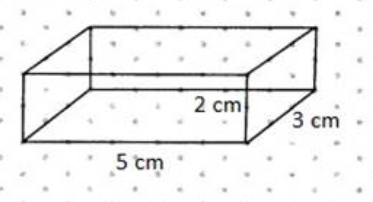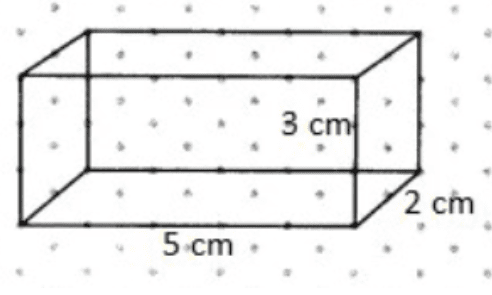3. Three cubes each with a 2 cm edge are placed side by side to form a cuboid. Sketch an oblique or isometric sketch of this cuboid.

Ans: Isometric Sketch

Isometric Sketching is a representation of an object in which all the three-dimensions are drawn at the full scale. It looks like an isometric projection. In this case, all the lines parallel to its major axes are measurable

Isometric sketch of three cubes each with 2 cm edge are placed side by side to form a cuboid is given below,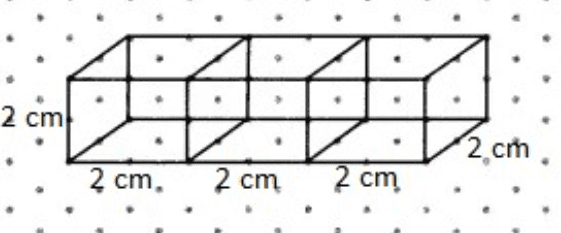Oblique sketch is a way of representing an object in pictorial form. Basically, the circles and angles parallel to the plane of projection are considered to be the true size and shape of the object while constructing oblique sketches.

Oblique sketch of three cubes each with 2 cm edge are placed side by side to form a cuboid is given below,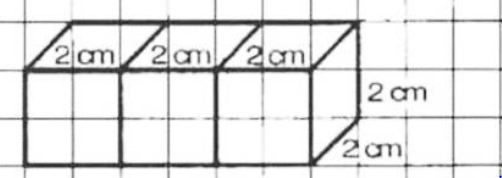4. Make an oblique sketch for each one of the given isometric shapes:

(i)

Ans: Oblique sketch is a way of representing an object in pictorial form. Basically, the circles and angles parallel to the plane of projection are considered to be the true size and shape of the object while constructing oblique sketches.

Oblique sketch of given sketch is given below,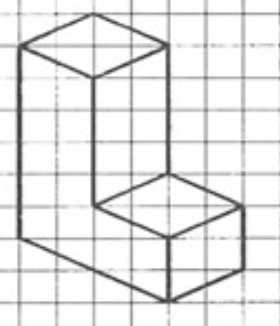(ii)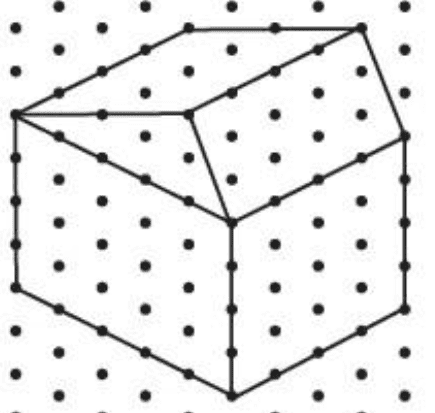Ans: Oblique sketch is a way of representing an object in pictorial form. Basically, the circles and angles parallel to the plane of projection are considered to be the true size and shape of the object while constructing oblique sketches.

Oblique sketch of given sketch is given below,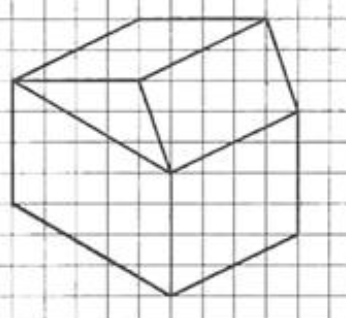5. Give

(i) An oblique sketch

(a) A cuboid of dimensions 5 cm, 3 cm and 2 cm.

Ans: Oblique sketch is a way of representing an object in pictorial form. Basically, the circles and angles parallel to the plane of projection are considered to be the true size and shape of the object while constructing oblique sketches.

Oblique sketch of given sketch is given below,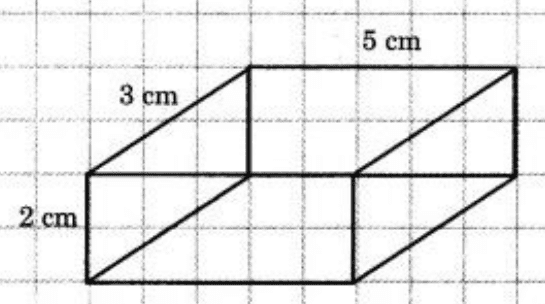(b) A cube with an edge 4 cm long.

Ans: Oblique sketch is a way of representing an object in pictorial form. Basically, the circles and angles parallel to the plane of projection are considered to be the true size and shape of the object while constructing oblique sketches.

Oblique sketch of given sketch is given below,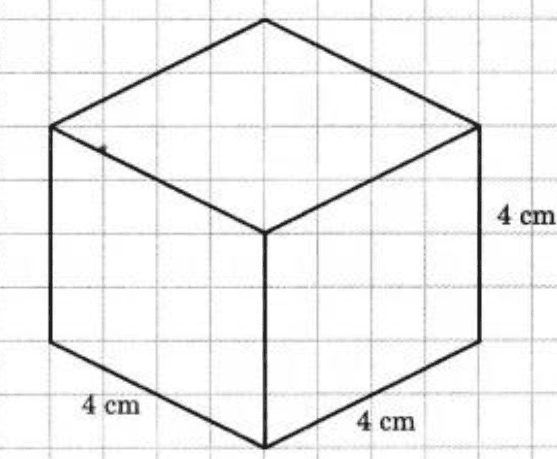(ii) An isometric sketch for each of the following:

(a) A cuboid of dimensions 5 cm, 3 cm and 2 cm.

Ans: Isometric Sketching is a representation of an object in which all the three-dimensions are drawn at the full scale. It looks like an isometric projection. In this case, all the lines parallel to its major axes are measurable

Isometric sketch of cuboid of dimensions 5 cm, 3 cm and 2 cm is given below,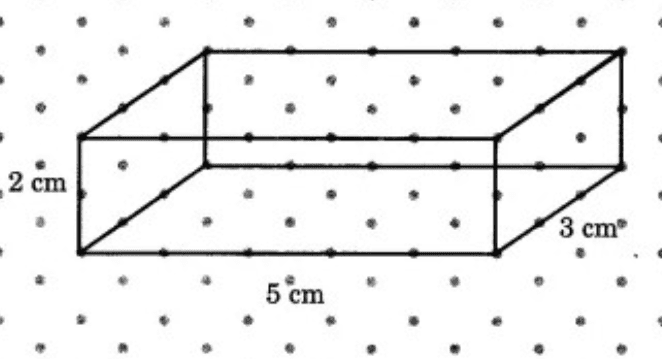(b) A cube with an edge 4 cm long.

Ans: Isometric Sketching is a representation of an object in which all the three-dimensions are drawn at the full scale. It looks like an isometric projection. In this case, all the lines parallel to its major axes are measurable.

Isometric sketch of cube with and edge 4 cm is given below,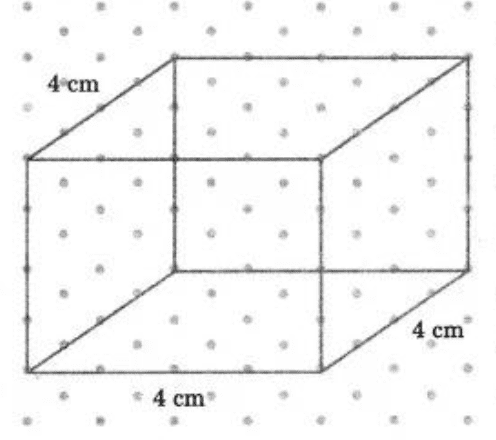## NCERT Solutions for Class 7 Maths Chapter 15 Visualising Solid Shapes Exercise 15.2

Opting for the NCERT solutions for Ex 15.2 Class 7 Maths is considered as the best option for the CBSE students when it comes to exam preparation. This chapter consists of many exercises. Out of which we have provided the Exercise 15.2 Class 7 Maths NCERT solutions on this page in PDF format. You can download this solution as per your convenience or you can study it directly from our website/ app online.

Vedantu in-house subject matter experts have solved the problems/ questions from the exercise with the utmost care and by following all the guidelines by CBSE. Class 7 students who are thorough with all the concepts from the Subject Visualising Solid Shapes textbook and quite well-versed with all the problems from the exercises given in it, then any student can easily score the highest possible marks in the final exam. With the help of this Class 7 Maths Chapter 15 Exercise 15.2 solutions, students can easily understand the pattern of questions that can be asked in the exam from this chapter and also learn the marks weightage of the chapter. So that they can prepare themselves accordingly for the final exam.

Besides these NCERT solutions for Class 7 Maths Chapter 15 Exercise 15.2, there are plenty of exercises in this chapter which contain innumerable questions as well. All these questions are solved/answered by our in-house subject experts as mentioned earlier. Hence all of these are bound to be of superior quality and anyone can refer to these during the time of exam preparation. In order to score the best possible marks in the class, it is really important to understand all the concepts of the textbooks and solve the problems from the exercises given next to it.

Do not delay any more. Download the NCERT solutions for Class 7 Maths Chapter 15 Exercise 15.2 from Vedantu website now for better exam preparation. If you have the Vedantu app in your phone, you can download the same through the app as well. The best part of these solutions is these can be accessed both online and offline as well.SHARETWEETSHARESUBSCRIBE